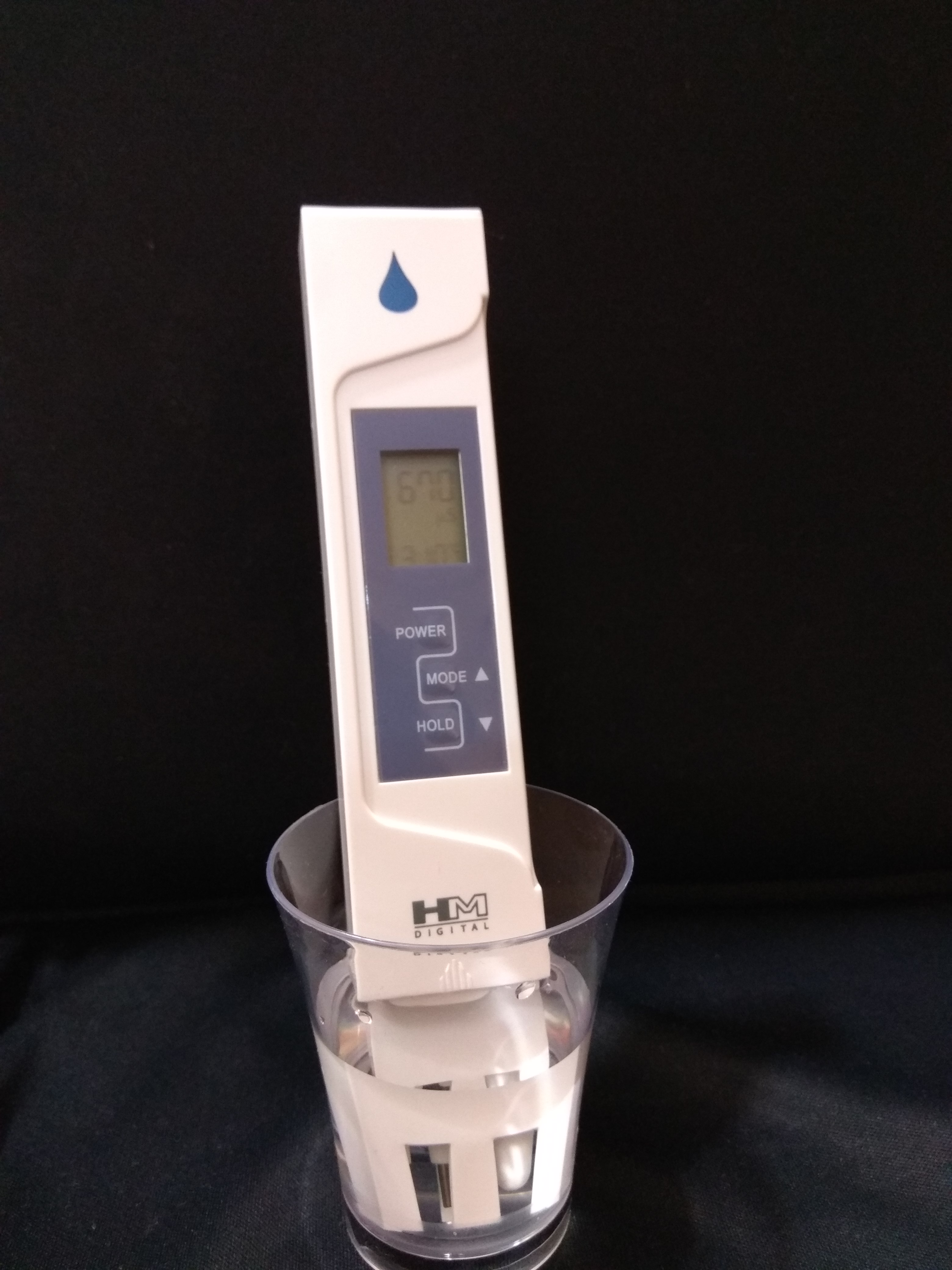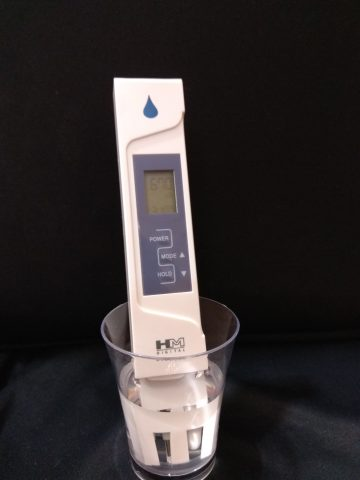# TDS vs EC meter – Which one to buy?

If you are a beginner in Hydroponics, it is certain, at some point, you would have had this question in your mind.

Should I buy an EC meter or a TDS meter?Using EC meters in Hydroponics

While the users are split on which one is better, it is good to know about both the options and decide which meter to buy. This post is exactly for that purpose, helping you decide what to buy but not before learning about them.

To understand TDS vs EC meter conundrum better, it helps to understand the science behind measuring salt concentration in hydroponics.

## Understanding Electrical Conductivity in Hydroponics

Hydroponics is all about feeding the right nutrient solution at the right concentration at the right time. The key here is right concentration. How do we know if the concentration we are providing is right or not. Meters! We have instruments that measure the concentration of salts in water and hence we can identify how much salt is a unit volume of water.

The principle behind measuring concentration is that salts dissociate into ions when dissolved in water and these ions conduct electricity. We all know pure water doesn’t conduct any electricity. We can say the measure of the electricity conducted is proportional to the ions present in the solution. This is called Conductivity of the solution. The conductivity of the solution is measured in Siemens/Meter. For the nutrient solutions, the measure is small and hence measured in mille siemems/centimeter (mS/cm) or microsiemens/cm (µS/cm)

So far so good. We understood what causes the solution to conduct electricity and also understood how it is measured.

The way the conductivity meters work is, there are two electrodes present at the bottom of the probe. These electrodes are separated by exactly 1 cm between them. An electrical charge is applied at one end and measured at the other end.

Based on the amount of electricity received on the other electrode the conductivity of the solution is calculated.

For example

Pure water doesn’t conduct any electricity at all so it will measure – 0 mS/cm.
Tap water has some amount of salt in it and it will conduct some electricity. Say 0.2 mS/cm.

In a world with only conductivity meters, no one talks about the amount of salts the solution contains. We only talk about the amount of electricity the salts present in the solution conduct.

Enter TDS Meters

## TDS – Total Dissolved Solids Explained

TDS stands for Totally dissolved solids. It means the quantity of solids that are dissolved in it. It is measured in PPM which is a shortform for Parts Per Million.

1 PPM of a salt means 1 part of the salt to million parts of water. Which essentially means 1milligram of salt in 1 liter of water.

1PPM = 1milligram in 1 liter of water.

But How do you find out how many milligrams are there in a solution without weighing it? This is where the fun begins.

TDS meters are actually EC meters inside but they also do a conversion from electrical conductivity to ppm. This is done by having a standard reference solution.

To convert EC to PPM, a standard reference solution such as sodium chloride is used. With a calculated quantity of weight dissolved in water and its EC is measured. Based on this the EC is measured with the known concentration.

## Various Standards in TDS Meter

For eg. 500 mg/liter of NACL solution measures 1mS/cm. This gives a conversion factor of 500.
Likewise, Potassium chloride is another reference solution that has a factor of 700.

And there is also a mixture of salts in the ratio 40:40:20 popularly known as 442.

If you observe closely, there are already 3 standards we discussed. It means that TDS meters from these standards respectively will measure 3 different readings for the same solution.

So the first question you need to ask when someone mentions their PPM readings is, which standard? And to make things worse, the most of the pH meters don’t have their standard written on them( Common sense?).

Next thing you know, you are already all over the internet looking for your pH meter’s data sheet. Not if you own an EC meter!

This is mainly the reason why an EC meter is preferred over a TDS meter. That said, it is not wrong to own a TDS meter. You can totally have a TDS meter and get good results as long as you know about the meter and its standard.